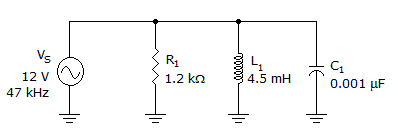# Electronics - RLC Circuits and Resonance - Discussion

### Discussion :: RLC Circuits and Resonance - General Questions (Q.No.30)

30.What is the current through the capacitor in the given circuit?

 [A]. 3.5 mA [B]. 5.5 mA [C]. 9 mA [D]. 11.4 mA

Explanation:

No answer description available for this question.

 Gaurav said: (Aug 6, 2011) Vc=I*Xc In parallel circuit Voltage is same at all the points. V=12V, Xc=3386.27. I=12/3386.27 I=3.53mA

 Tommy said: (Oct 18, 2013) How do you get Xc = 3386.27. Thanks.

 Om Prakash Bharti said: (Jan 15, 2014) Xc = 1/wc = 1/2*pi*f*C. = 1/2*3.14*47000*0.001*10^-6. = 3386.27.

 Afash said: (Jan 16, 2014) Very simple: Xc = 1/2*pi*f*C. So, Xc = 1000000/2*Pi*47*1000*0.001. So you will get 3386.275. Then I = 12/3386.275. I=3.543mA.